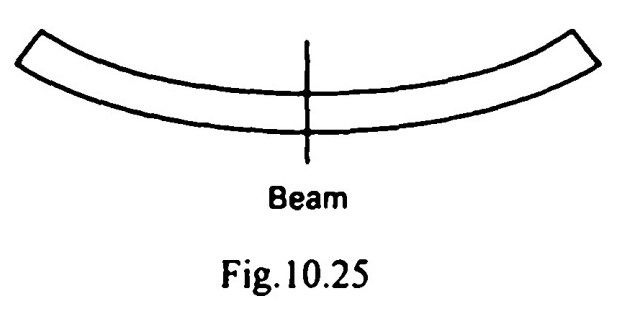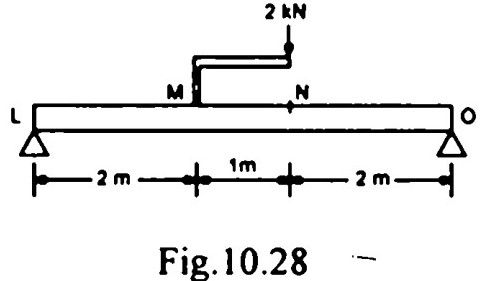top of pageSearch

# Strength of Materials(201-220)

Updated: Apr 28, 2020

201. In a stressed body, an elementary cube of material is taken at a point with its faces perpendicular to x and y reference axes. Tensile stresses equal to 15 kN/cm² and 9kN/cm² are observed on these respective faces. They are also accompanied by shear stresses equal to 4 kN/cm². The magnitude of the principal stresses at the point are 17 kN/cm² tensile and 7 kN/cm² tensile

202. In. a rectangular element subjected to like principal tensile stresses p and p in two mutually perpendicular directions x and y, the maximum shear stress would occur along the planes at 45° and 135° to the y direction

203. When a mild steel specimen fails in a torsion-test, the fracture looks like204. At a point in a structure, there ate two mutually perpendicular tensile stresses of 800 kg/cm² and 400 kg/cm². If the Poisson's ratio is n = 0.25, what would "be the equivalent stress in simple tension according to Maximum Principal Strain Theory?

700 kg/cm²

205.For a square-sectioned beam bent as shown in Fig, the exaggerated view

of the deformed cross-section is206.According to maximum shear stress failure criterion, yielding in material

occurs when maximum shear stress = 1/2 stress

207.

1. For a section having one axis of symmetry, the shear centre does not concincide with the centroid but lies on the axis of symmetry.

2. If a load passes through the shear centre, then there will be only bending in the cross section and no twisting.

208.In a thin walled T-section, the shear centre C is located at the point shown in209.The bending moment diagram of the beam shown in Fig. is210.The SFD and BMD for a beam are shown in 10.30(a) and 10.30(b). The correspond211. A bar of circular cross section varies uniformly from a cross section 2D to D. If

extension of the bar is calculated treating it as a bar of average diameter, then the

percentage error will be 10

212.The length, coefficient of thermal expansion and Young's modulus of bar

'A' are twice that of bar ' B '. If the temperature of both bars is increased by

the same amount while preventing any expansion, then the ratio of stress

developed in bar A to that in bar B will be 4

213. The side AD of the square block ABCD as shown in Fig. is fixed at the base and it is under a state of simple shear causing shear stress x and shear strain φ , whereφ/2

214. The lists given below refer to a bar of length l cross sectional area A, Young's

modulus E. Poisson's ratio µ and subjected to axial stress p. Match List I with List II and select the correct answer using the codes given below the lists :215. If all the dimensions of a prismatic bar of square cross section suspended freely from the ceiling of a roof are doubled, then the total elongation produced by its own weight will increase four times

216. On an element shown in Fig. 10.33, the stresses are (in MPa)The radius of Mohr's circle and principal

stresses σ, σ are (in MPa)

50 20 120

217.

1. When the structural member is subjected to uniaxial loading, the shear stress is zero on a plane where the normal stress is maximum.

2. In a member subjected to uniaxial loading, the normal stress on the

planes of maximum shear stress is less than the maximum

218. A certain steel has proportionality limit of 3000 kg/cm² in simple tension. It is

subjected to principal stresses of 1200 kg/cm² (tensile), 600 kg/cm² (tensile) and

300 kg/cm² (compressive). What would be the factor of safety according to maximum shear stress theory? 2.00

219.

A. Shear centre - Twisting B. Principal plane- Shear stress C. Fixed end - Slope D. Middle third rule - Tension

220.The maximum deflection of a fixed beam carrying a central load W is equal to